# Capacitors in Parallel Formula

Capacitors in Parallel Formula

In electric circuits, it is often possible to replace a group of capacitors with a single, equivalent capacitor. The equivalent capacitance of a number of capacitors in parallel is the sum of the individual capacitances. The unit of capacitance is the Farad (F), which is equal to a Coulomb per Volt (1 F = 1 C/V), though most electronic circuits use much smaller capacitors. Picofarad (1 pF = 10-12 F), nanofarad (1 nF = 10-9 F), and microfarad (1 µF = 10-6 F) capacitors are common.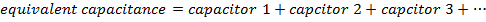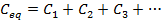Ceq = equivalent capacitance (F or smaller units)

C1 = capacitance of first capacitor (F)

C2 = capacitance of second capacitor (F)

C3 = capacitance of third capacitor (F)

Capacitors in Parallel Formula Questions:

1) What is the equivalent capacitance of a 400.0 pF, a 200.0 pF, and a 50.0 pF capacitor connected in parallel?

Answer: The capacitances are all expressed in picofarads, and so there is no need to change their units. The equivalent capacitance can be found in picofarads using the formula: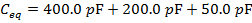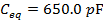The equivalent capacitance of the 400.0 pF, 200.0 pF, and 50.0 pF capacitors in parallel is 650.0 pF.

2) Two capacitors are connected in parallel in an electric circuit. Their capacitances are 500.0 nF, and 2.00 µF. What is the equivalent capacitance?

Answer: The capacitance values are expressed using different units. The first step to finding the equivalent capacitance is to convert these to a common unit. It is not necessary to convert them all to Farads. One of the values can be converted to the same unit as the other. In this solution, the values will be converted to microfarads.

If C1 = 500.0 nF, and C2 = 2.00 µF, then:

C1 = 500.0 nF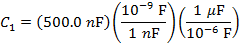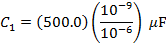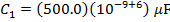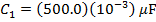C1 = 0.500 µF

The equivalent capacitance can now be found using the formula: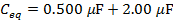Ceq = 2.50 µF

The equivalent capacitance of the 500.0 nF and 2.00 µF capacitors in parallel is 2.50 µF.